The factory

The factory z can complete an order in 12 days, factory p in 18 days. For how many days does an order completed when the first two days work only the factory z and then both factories?

Result

t =  8 d

Solution:

t/12 + (t-2)/18=1

5t = 40

t = 8

Calculated by our simple equation calculator.

Leave us a comment of this math problem and its solution (i.e. if it is still somewhat unclear...):Be the first to comment!To solve this verbal math problem are needed these knowledge from mathematics:

Looking for help with calculating harmonic mean? Looking for a statistical calculator? Do you have a linear equation or system of equations and looking for its solution? Or do you have quadratic equation?

Next similar math problems:

1. Working together on projectTwo employees working on the project. First make it alone for 20 days the second for 24 days. How many days will take to complete project if 5 days they work together then first will take two days holiday and after returning they completes a project toget
2. Workers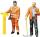Two workers fulfill a certain task when working together for 5 days, the first if he worked himself done work in 13 days. How many days will do whole job a second worker?
3. Construction of roadFor the construction of the road dam, it is supposed to transport six trucks a total of 18 days. After three days, two more cars were sent to the construction site. How long will it take to carry the material?
4. Dwarfs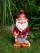Four dwarfs would prepare firewood for Snow White in580 minutes. After an hour and a half they recruit friends so that finished preparing the wood for 280 minutes. How many dwarfs they recruited?
5. PumpingThe tank is filled with a larger pump in 12 hours and with scond pump in 15 hours. For how long does the tank fill when both pumps are switched on at the same time?
6. Hectoliters of waterThe pool has a total of 126 hectoliters of water. The first pump draws 2.1 liters of water per second. A second pump pumps 3.5 liters of water per second. How long will it take both pumps to drain four-fifths of the water at the same time?
7. NovakNovak needed to dig up three of the same pit in the garden. The first pit dug father alone for 15 hours. His second dig son helped him and it did that in six hours. The third pit dug son himself. How long it took him?
8. Sprayers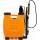The vineyard can be sprayed first sprayer in 5 hours and with the second in 8 hours. How long would it take if we use sprayers both at once?
9. Machines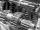In the workshop are three machines. The first is treated 250 parts for 4.7 hours, the second is treated 300 parts for 1.9 hours and the third is treated 230 parts for 4.6 hours. How long will take to make 3100 parts if worked all three machines at the.
10. Two pumps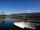The first pump fills the tank in 24 hours second pump in another 40 hours. For how long will take fill the tank if operate both pumps at the same time?
11. Tanker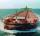An oil tanker can be emptied by the first pump in 4.9 hours. Second pump can empty an tanker in 8 hours. If the first pump started at 4:00 and second 1.4 hour later, at what time will the tanker be empty?
12. Harvesters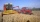The first harvester will harvest the grain from the field in 20 hours, the second, the more powerful in 10 hours. How long will take harvest this field by both harvesters, if the second harvester has to be set up first and this takes 2 hours? The first har
13. Simple equationSolve for x: 3(x + 2) = x - 18
14. Unknown numberIdentify unknown number which 1/5 is 40 greater than one tenth of that number.
15. Pumps A and BPump A fill the tank for 12 minutes, pump B for 24 minutes. How long will take to fill the tank if he is only three minutes works A and then both pumps A and B?
16. EquationSolve the equation: 1/2-2/8 = 1/10; Write the result as a decimal number.
17. Six yearsIn six years Jan will be twice as old as he was six years ago. How old is he?